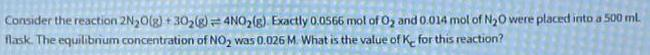Question:

# Consider the reaction 2N₂O(g) + 30₂(g) = 4NO₂(g) Exactly

Last updated: 8/10/2022Consider the reaction 2N₂O(g) + 30₂(g) = 4NO₂(g) Exactly 0.0566 mol of O₂ and 0.014 mol of N₂0 were placed into a 500 ml. flask. The equilibrium concentration of NO₂ was 0.026 M. What is the value of K for this reaction?